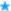International Journal of                                                 Numerical Analysis & Modeling
 ISSN 1705-5105Aims and ScopesEditorial BoardContentsTo AuthorsTeX Style filesSubscriptionContacts
 "For the things of this world cannot be made known without a knowledge of mathematics" -----Roger Bacon
AIMS & SCOPES

IJNAM  is covered in Science Citation Index-Expanded (SCIE) and                          Current Contents/Physical, Chemical & Earth Sciences (CC/PC&ES)                       Indexed  both in Mathematics and  Applied Mathematics with the impact factor 0.808 (2018JCR)

 The journal is directed to the broad spectrum of researchers in numerical methods throughout science and engineering, and publishes high quality original papers in all fields of numerical analysis and mathematical modeling including: numerical differential equations, scientific computing, linear algebra, control, optimization, and related areas of engineering and scientific applications. The journal welcomes the contribution of original developments of numerical methods, mathematical analysis leading to better understanding of the existing algorithms, and applications of numerical techniques to real engineering and scientific problems. Rigorous studies of the convergence of algorithms, their accuracy and stability, and their computational complexity are appropriate for this journal. Papers addressing new numerical algorithms and techniques, demonstrating the potential of some novel ideas, describing experiments involving new models and simulations for practical problems are also suitable topics for the journal. The journal welcomes survey articles which summarize state of art knowledge and present open problems of particular numerical techniques and mathematical models. ©ISCI
 ==   Northwestern Polytechnical  University and Institute for Scientific Computing  and Information   since 2004 ==